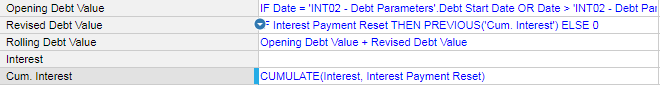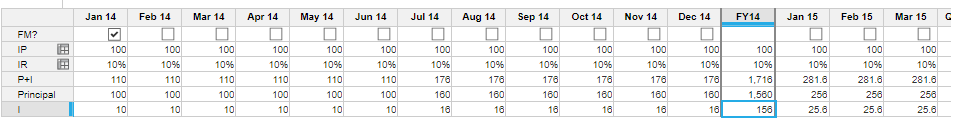# Circular ReferencesHi,

I am having problems with what I think should be a fairly simple calculation but I'm not sure what I'm doing wrong.  I have a debt value.  Interest is calculated on that debt value.  Every 6 months, the cumulative interest is added to the debt value and the interest for the next 6 months is calculated on the new debt balance.

In the screenshot below, the opening debt value holds the original amount.  Revised debt value returns the cumulative interest at the required date.  Rolling adds them together.  The circular reference comes when I try to calculate the Interest from the rolling debt value.  Any ideas how to resolve this would be gratefully received!Tagged:

•Hi Sally,

Try creating a separate line item that performs the PREVIOUS('Cumulate. Interest') Calc, that may do the trick. If that doesn't work could you provide a screenshot with a view of the dimensions and rest of the line items?

Thanks!

Griffin

•Hi Sally,Starting Period(FM?) - Jan 14

Initial Principal(IP) - 100

Interest Rate(IR) - 10%

Calculation:

Interest for the principal 100.0 for 6 months at 10% rate is 60.0

New Principal after 6 month is 100+60 = 160

Interest for the new principal 160.0 for 6 months at 10% is 96

New Principal after 6 month is 160+96 = 256

On jan 14, the principal amount is 100. On Jul 14 the principal amount become 160. On Jan 15 the principal become 256.

The formulas as below,

FM? :  Input

IP : input

IR : Input

P+I

IF FM? THEN IP * (1 + IR) ELSE IF MONTH(START()) = 1 OR MONTH(START()) = 7 THEN (POST('P+I', 1) / (1 + IR) + (POST('P+I', 1) - POST('P+I', 1) / (1 + IR)) * 6) * (1 + IR) ELSE POST('P+I', 1) / (1 + IR) * (1 + IR)

Principal : 'P+I' / (1 + IR)

I : 'P+I' - Principal

~Vignesh

•Hi Vignesh,

I was trying to implement the below solution you suggested, but problem is my module don't use time dimension.

so, start() and POST() functions are not applicable,

I Just want to get the formula as below:

Line Item1 = Total

Total = Line item1+Line item2

I am getting circular reference error here.

Thanks